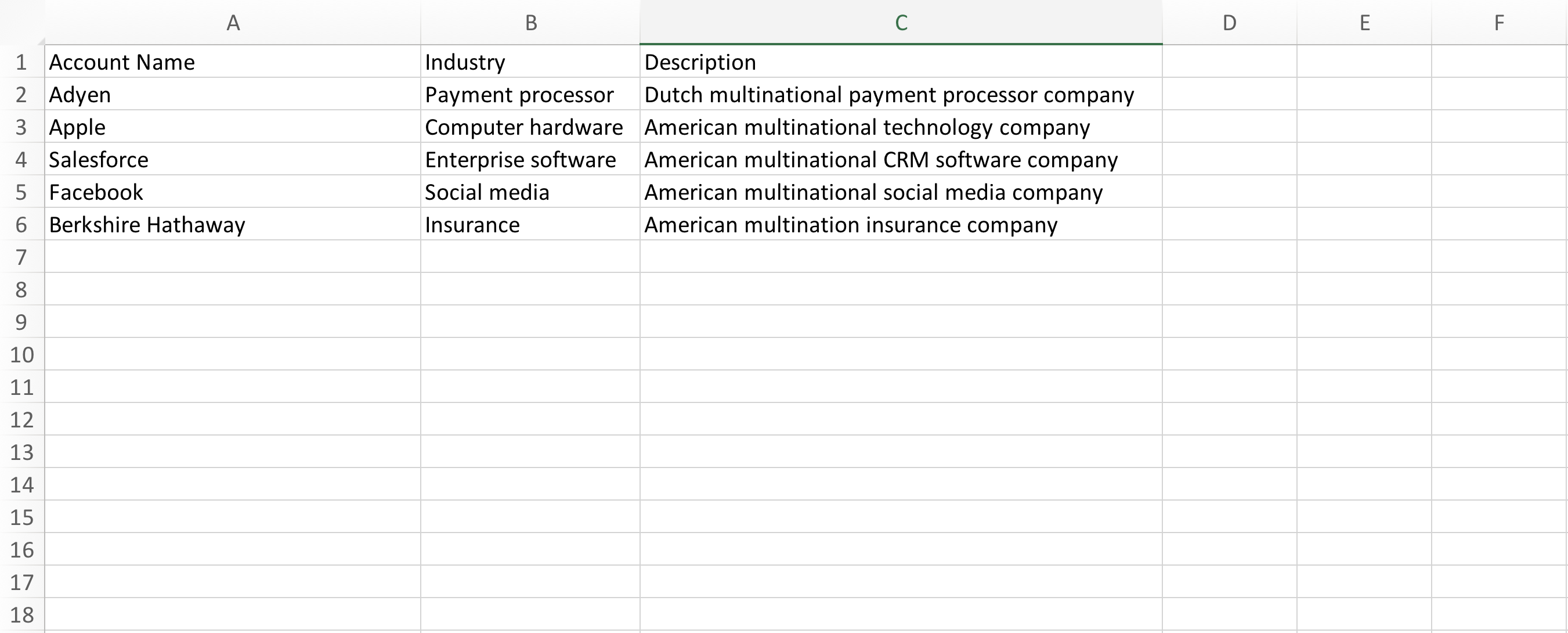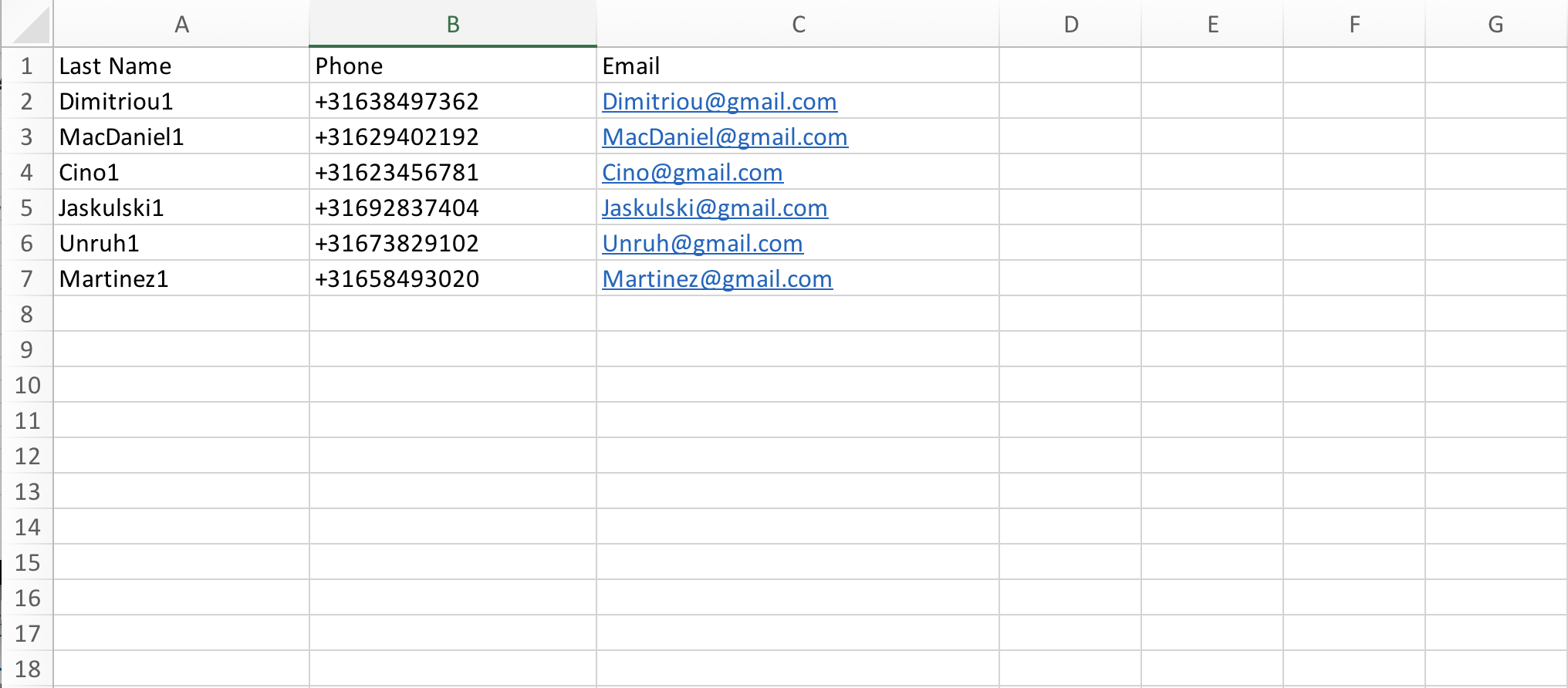In this article, we give a quick overview of the data importing functionality.

let's start with importing new accounts. First we structure the data in a sheet where the first row represent the object names and the rows below that represents the data we wish to import. See below for an example.Second, we save the file as a csv type and follow the steps:

When there are no errors resulting from the import, the new account will show up on the account tab. Let's do another example and import contacts. First we create a csv type file:Then we follow the steps below to import the data: